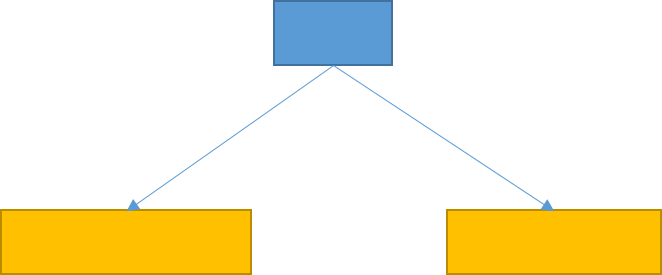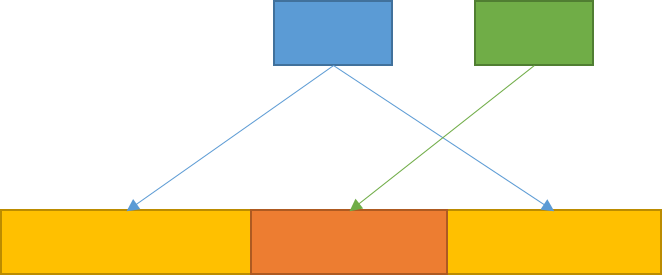Luogu 1514 引水入城 （搜索，动态规划）

Description2 5
9 1 5 4 3
8 7 6 1 2

1
1

解决思路代码

#include<iostream>
#include<cstdio>
#include<cstdlib>
#include<cstring>
#include<algorithm>
#include<queue>
using namespace std;

const int maxN=600;
const int F1={0,1,-1,0,0};//两个F数组分别是在bfs搜索时的四个方向
const int F2={0,0,0,1,-1};
const int inf=2147483647;

class Range//区间
{
public:
int l,r;
};

class Pos//位置，bfs里要用
{
public:
int x,y;
};

bool operator <(Range A,Range B)//之前贪心算法时要用到排序
{
return (A.l<B.l)||((A.l==B.l)&&(A.r>B.r));
}

int n,m;
int Rangecnt=0;//统计总共找到了多少个可行区间
int Height[maxN][maxN];//海拔
bool vis[maxN][maxN];//bfs中标记是否走过
bool is_cover[maxN];//标记最后一排城市是否走过，方便判断无解
Range R[maxN];//区间
queue<Pos> Q;//bfs队列
int F[maxN];//最后求最少区间覆盖时动态规划

void bfs(int st);

int main()
{
memset(is_cover,0,sizeof(is_cover));//初始化
Rangecnt=0;
scanf("%d%d",&n,&m);
for (int i=1;i<=n;i++)
for (int j=1;j<=m;j++)//读入海拔
scanf("%d",&Height[i][j]);
Height=0;//为了方便判断，在第一个城市左边和第二个城市右边加入高度为0的城市
Height[m+1]=0;
for (int i=1;i<=m;i++)//对于第一行中满足比它两边都高的城市进行bfs搜索区间
if ((Height[i]>=Height[i-1])&&(Height[i]>=Height[i+1]))
bfs(i);
int sum=0;//sum用来统计总共覆盖了多少城市，如果sum!=m，则无解
for (int i=1;i<=m;i++)
sum+=(int)(is_cover[i]);
if (sum<m)
{
printf("0\n%d\n",m-sum);
return 0;
}
sort(&R,&R[Rangecnt+1]);//其实这个排序是不必要的
memset(F,126,sizeof(F));
F=0;
for (int i=1;i<=Rangecnt;i++)//动态规划求解最少区间覆盖
for (int j=R[i].l;j<=R[i].r;j++)
F[j]=min(F[j],F[R[i].l-1]+1);
printf("1\n%d\n",F[m]);
return 0;
}

void bfs(int st)//bfs求解
{
memset(vis,0,sizeof(vis));
Pos init;
init.x=1;
init.y=st;
vis[st]=1;
while (!Q.empty())
Q.pop();
Q.push(init);
do
{
Pos u=Q.front();
Q.pop();
for (int i=1;i<=4;i++)
{
int x=u.x+F1[i];
int y=u.y+F2[i];
if ((x<=n)&&(x>=1)&&(y<=m)&&(y>=1)&&(Height[u.x][u.y]>Height[x][y])&&(vis[x][y]==0))
{
Q.push((Pos){x,y});
vis[x][y]=1;
}
}
}
while (!Q.empty());
int l=-1,r=-1;//记录覆盖区间
for (int i=1;i<=m;i++)
{
if ((vis[n][i]==1)&&(l==-1))
l=i;
if ((vis[n][i]==0)&&(l!=-1)&&(r==-1))
r=i-1;
if (vis[n][i]==1)//同时更新is_cover数组
is_cover[i]=1;
}
if (l==-1)//可能出现当前选择的最上面一排的城市无法到达最下面一排，此时直接退出
return;
if (r==-1)//当r==-1说明覆盖到了最后一个城市
r=m;
Rangecnt++;
R[Rangecnt].l=l;
R[Rangecnt].r=r;
return;
}
posted @ 2017-09-03 12:18 SYCstudio 阅读(...) 评论(...) 编辑 收藏